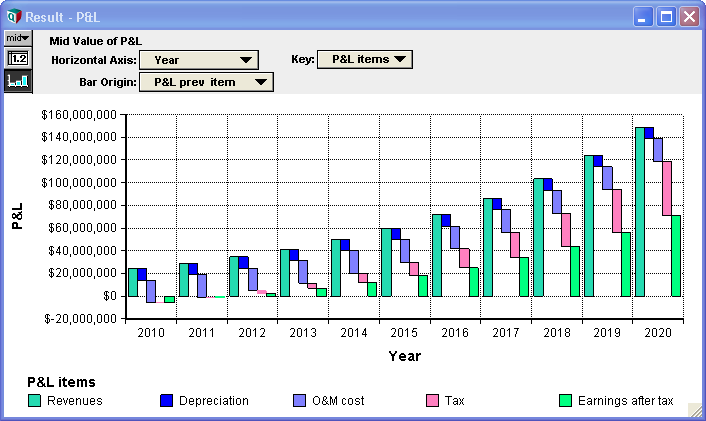# Waterfall Charts

(diff) ← Older revision | Latest revision (diff) | Newer revision → (diff)A waterfall chart is a type of bar chart, similar to a stacked bar chart except that bars don't overlap and the origin of each bar starts where the previous bar ended, allowing negative values to be readily depicted. It is often used because most graphing packages don't handle negative value intelligently when plotting stacked bar charts. Analytica's standard stacked bar chart does handle negative values intelligently, so it does provide a good alternative to the waterfall chart. This page provides instructions for plotting a standard waterfall-variety of bar chart, as depicted in the diagram above.

The example used in the above chart can be downloaded here: Waterfall chart example.ana

The waterfall chart is in the "P&L" result graph.

## How its done

To create the chart, you will plot a bar chart with 0% bar overlap. The end of each bar is the cumulative value of the result across the key index, the same way it would be in a stacked bar chart. The origin of each bar is the previous cumulative value along the key index -- i.e., where the previous bar left off.

In the example, we wish to create a waterfall of P_L_items ("P&L items"), which is a self-indexed array, with the self-index P_L_items serving as the graph key.

To be more general, in what follows, we'll refer to the value to be plotted as X and the key-index as K. Our waterfall graph will appear in a new variable, Waterfall_X, defined as:

Cumulate(X, K)

Next, we need to compute the origin for each bar. We'll compute this in a variable named Waterfall_prev defined as:

if @K = 1 then 0 else Waterfall_X[@K = @K - 1]

This states that each bar will start where the previous bar left off, with the first bar in each series starting at 0.

Show the result graph for Waterfall_X and press the XY button on the top-right corner. Check Use another variable and add Waterfall_prev, then press OK to close the XY comparison sources dialog.

Pivot the graph so that K is the graph key, and your time dimension is on the horizontal axis.

Select 'Result → Graph Setup.... Set the Line Style to solid bar, place a check box on Variable origin, uncheck 'Swap horizontal and vertical, and enter Bar overlap = 0%. Press Apply.